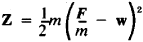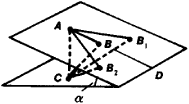Least Constraint, Principle of

Least Constraint, Principle of

one of the variational principles of mechanics, according to which for a mechanical system with ideal constraints, of all kinematically possible motions—that is, of all motions permitted by the constraints—beginning from a given position and with given initial velocities, the true motion will be that for which the “constraint” Z is the least at each point in time. This principle was established by K. Gauss in 1829.

The physical quantity called constraint is introduced in the following manner. A free particle of mass m will, when acted upon by a given force F, have acceleration F/m; however, if constraints are superimposed on the particle, its acceleration for the same force F will be equal to some other quantity w. Then the deviation of the point from free motion brought about by the action of the constraint will depend on the difference between these accelerations—that is, on F/m - w. The quantity Z, which is proportional to the square of this difference, is called the constraint. For one pointbut for a mechanical system Z is equal to the sum of these quantities.

Let us consider, for example, a point that begins to move along a smooth inclined plane from position A without initial velocity (see Figure 1). Any movement AB, AB1, AB2, … in this plane with some accelerations w, w1 w2,… is kinematically possible for it; however, in free fall the point would accomplish the displacement AC along the vertical with acceleration g. Then the deviations of the point from free motion will be depicted by segments CB, CB1 CB2, … , the shortest of which would be segment CB, which is perpendicular to the inclined plane. Consequently the constraint Z, which is proportional to the squares of CB, CB1 CB2, …, will be smallest for motion along the line of least slope AD. This will be the point’s true motion, which proceeds with an acceleration w = g sin α.Figure 1

The principle of least constraint is used to construct equations of the motion of mechanical systems and to study the properties of these motions.

Site: Follow: Share:
Open / Close